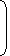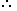# Tricks to Solve Problems on Square Root & Cube Root## Square Root

If x2 = y,

we say that the square root of y is x and we write y = (+/-)x

Thus, 4 = 2, -2

9 = 3, -3

196 = 14, -14

## Cube Root:

The cube root of a given number x is the number whose cube is x.

We, denote the cube root of x by 3√x.

Thus,  3√8 = 2 x 2 x 2 = 2,

3√343 = 7 x 7 x 7 = 7 etc.

Note:

• 1. xy = x    x    y

2.
 √x √y
= x = x x y = xy .
y y y y

Problems on Square Root & Cube Root

The cube root of .000216 is:
 A. 0.6 B. 0.06 C. 77 D. 87

Explanation:

 (.000216)1/3 =2161/3 106
 =6 x 6 x 61/3 102 x 102 x 102
 = 6 102
 = 6 100

= 0.06

1.5625 = ?
 A. 1.05 B. 1.25 C. 1.45 D. 1.55

Explanation:

```   1|1.5625( 1.25
|1
|-------
22| 56
| 44
|-------
245| 1225
| 1225
|-------
|    X
|-------
```1.5625 = 1.25.

Correct! Wrong!

Correct! Wrong!

Correct! Wrong!

Correct! Wrong!

#### The square root of 64009 is:

Correct! Wrong!

QUIZ ON SQUARE ROOT & CUBEROOT
great job!!!!!!!